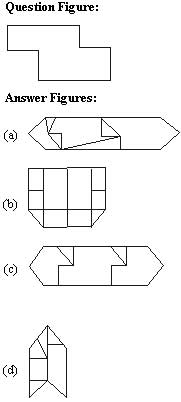## Subject: Reasioning

1. Bahu is Rahim’s neighbour and his house is 200 metres away in the north west direction. Joseph is Rahim’s neighbour and his house is located 200 metres away in the south west direction. Gopal is Joseph’s neighbour and he stays 200 metres away in the south east direction. Roy is Gopal’s neighbour and his house is located 200 metres away in the north east direction. Then where is the position of Roy’s house in relation to Babu’s ?

(a) South east
(b) South west
(c) North
(d) North east

2. A group of friends are sitting in an arrangement one each at the corner of an octagon. All are facing the centre. Mahima is sitting. diagonally opposite Rama, who is on Sushma’s right. Ravi is next to Sushma and opposite Girdhar, who is on Chandra’s left. Savitri is not on Mahima’s right but opposite Shalini. Who is on Shalini’s right?

(a) Ravi
(b) Mahima
(c) Girdhar
(d) Rama

3. A cube has the following figures drawn on its five faces. The top surface is blank. The ellipse is between the cross and the triangle. The square is on the right of the triangle. The ellipse and the square are opposite to each other. Which face is the circle on?

(a) On the top
(b) Opposite to ellipse
(c) Opposite to triangle
(d) At the bottom

Directions (4 – 11) : In each of the following questions, select the related word/letters/ number/ figure from the given alternatives.

4. Fox : Cunning: : Rabbit:,?

(a) Courageous
(b) Dangerous
(c) Timid
(d) Ferocious

5. Flexible : Rigid : Confidence : ?

(a) Diffidence
(b) Indifference
(c) Cowardice
(d) Scare

6. AZCX : BYDW :: HQJO : ?

(a) GRFP
(b) IPKM
(c) IPKN
(d) GRJP

7. QIOK : MMKO :: YAWC : ?

(a) USGA
(b) UESG
(c) VUES
(d) SUEG

8. ABC/F : BCD/I :: CDE/L : ?

(a) DEF/O
(b) DEF/N
(c) EDF/O
(d) DEF/M

9. 1 : 8 :: 27 : ?

(a) 37
(b) 47
(c) 57
(d) 64

10. 24 : 126 :: 48 : ?

(a) 433
(b) 192
(c) 240
(d) 344

11. 987 : IHG :: 654 : ?

(a) FDE
(b) FED
(c) EFD
(d) DEF

Directions (12-18): In each of the following questions field the odd word/letters/number/figure from the given responses.

12.

(a) Room
(b) Chamber
(c) Veranda
(d) Cabin

13.

(a) Mouth Organ
(b) Electric Guitar
(c) Keyboard
(d) Sonata

14.

(a) A
(b) 1
(c) D
(d) E

15.

(a) RNMP
(b) JFEH’
(c) RPOQ
(d) HDCF

16.

(a) AbcdE
(b) IfghO
(c) ApqrL
(d) UImnE

17.

(a) 6243
(b) 2643
(c) 8465
(d) 4867

18.

(a) 49 – 33
(b) 62 – 46
(c) 83 – 67
(d) 70 – 55

19. From amongst the given alternatives, select the one in which the set of numbers is most like the set of numbers given below:

(6, 14,30)

(a) 4, 16, 28
(b) 7, 12, 22
(c) 6, 12, 22
(d) 5, 12, 20

20. Which one of the given responses would be a meaningful order of the following words ? A. Family B. Community C. Member D. Locality E. Country

(a) C, A, D, B, E
(b) C, A, B, D, E
(c) C, A, B, E, D
(d) C, A, D, E, B

21. Arrange the following words ac­cording to the dictionary:

A. TORTOISE
B. TORONTO
C. TORPED
D. TORUS
E. TORSEL

(a) B, E, C, A, D
(b) B, E, C, D, A
(c) B, C, E, A, D
(d) B, C, E, D, A

22. Which set of letters when sequentially placed at the gaps in the given letter series shall complete it ?
_a_aaaba_ ba ab

(a) abaaaa
(b) abaaba
(c) aababa
(d) ababaa

Directions (23-27): In each of the following questions, find the missing number /letters/figure from the given responses :

23. a, r, c, s, e, t, g, __, __,

(a) x, z
(b) u, i
(c) w, y
(d) v, b

24. (?), PSVYB, EHKNQ, TWZCF, ILORU

(a) B, E, H, K, N
(b) A, D, G, J, M
(c) S, V, Y, B, E
(d) Z, C, F, I, L

25. 0, 4, 18, 48, ?, 180

(a) 58
(b) 68
(c) 84
(d) 100

26. 36, 28, 24, 22, ?

(a) 18
(b) 19
(c) 21
(d) 22

27. 7, 9, 1.3, 21, 37, ?

(a) 58
(b) 63
(c) 69
(d) 72

28. Select the number which does NOT belong to the given series 232, 343,
454, 564, 676

(a) 676
(b) 454
(c) 343
(d) 564

29. A bus left with some definite number of passengers. At the first stop,half the passengers left the bus and 35 boarded the bus. At the second stop of the passengers left and 40 boarded the bus. Then, the bus moved with 80 passengers towards its destination without stopping any­where. How many passengers were there originally ?

(a) 25
(b) 30
(c) 40
(d) 50

30. If the day, after tomorrow is Sunday, what day was tomorrow’s day before yesterday ?

(a) Friday
(b) Thursday
(c) Monday
(d) Tuesday

31. A man is 3 years older than his wife and four times as old as his son. If the son becomes 15 years old after 3 years, what is the present age of the wife ?

(a) 60 years
(b) 51 years
(c) 48 years
(d) 45 years

32. X and Y are brothers. R is the father of Y. S is the brother of T and maternal uncle of X. What is T to R?

(a) Mother
(b) Wife
(c) Sister
(d) Brother

33. Suresh is 7 ranks ahead of Ashok in the class of 39 students. If
Ashok’s rank is 17th from the last, what is Suresh’s rank from the start?

(a) 16th
(b) 23th
(c) 24th
(d) 15th

34. A word/set of letters given in capital letters is followed by four answer words. Out of these only one cannot be formed by using the letters of the given word/set of letters. Find out that word INDETERMINATE

(a) DETERMINE
(b) RETINUE
(c) REMINDER
(d) RETINA

35. A group of alphabets are given with each being assigned a numerical code. These have to be unscrambled into a meaningful word and the correct code so obtained may be indicated from the given responses ?

R A H K S
1 2 3 4 5

(a) 51234
(b) 54213
(c) 53214
(d) 53124

36. A statement is given followed by two assumptions, (a) and (b). You have to consider the statement to be true, even if it seems to be at variance from commonly known facts. You are to decide which of the given assumptions can definitely be drawn from the given statement. Indicate your answer.

Statement: Theoretical education does not bring in economic
advancement and it lends to a steady loss of confidence and money in the
country.

Assumptions:
(a) There is close relationship between development of confidence and
economic development.
(b) Theoretical education makes priceless contribution for development of
confidence.

(a) Only 1 is implicit
(b) Only 2 is implicit
(c) Both 1 and 2 are implicit
(d) Both 1 and 2 are not implicit

37. Two statements are given followed by four conclusions, I, II, III and IV. You have to consider the statements to be true, even if they seem to be at variance from commonly known facts. You are to decide which of the given conclusions can definitely be drawn from the given statements. Indicate your answer.

Statements:
(A) No cow is a chair.
(B) All chairs are tables.

Conclusions:
I. Some tables are chairs.
II. Some tables are cows.
III. Some chairs are cows.
IV. No table is a cow

(a) Either II or III follow
(b) Either II or IV follow
(c) Only I follows
(d) All conclusions follow

38. If HONESTY is written as 5132468 and POVERTY as 7192068, how is HORSE written in a certain code?

(a) 50124
(b) 51042
(c) 51024
(d) 52014

39. In a certain code SISTER is written as RHRSDQ. How is UNCLE written in that code?

(a) TMBKD
(b) TBMKD
(c) TVBOD
(d) TMKBD

40. If 841 = 3, 633 = 5, 425 = 7 then 217 = ?

(a) 6
(b) 7
(c) 8
(d) 9

41. The following equations follow a common property. Find out the correct value to complete D : A=51(714)14 : B = 61 (915) 15; C = 71 (1136) 16: D = 81 (?) 17

(a) (1377)
(b) (1378)
(c) (1356)
(d) (1346)

42. After interchanging ÷ and =, 2 and 3 which one of the following statements becomes correct ?

(a) 15 = 2 = 3
(b) 5 ÷ 15 = 2
(c) 2 = 15 ÷ 3
(d) 3 = 2 ÷ 15

43. 25 * 2 * 6 = 4 * 11 * 0

Which set of symbols can replace * ?

(a) ×, –, ×, +
(b) +, –, ×, +
(c) x, +, ×, –
(d) x, +, +, ×

44. Find the missing number from the given responses

(a) 4
(b) 5
(c) 3
(d) 6

45. Peter walked 8 kms. west and turned right and walked 3 kms. The again he turned right and walked 12 kms. How far is he from the starting point ?

(a) 7
(b) 8
(c) 4
(d) 5

46. Choose the correct figure that represents the given relation : Blue eyed, females, doctors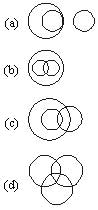47. Among the four answer figures, which figure can be formed from the
cutpieces given below in the question figure ?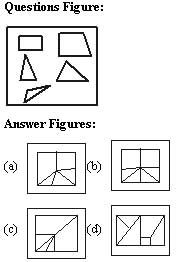48. If the mirror is placed on the line LM, then which of the answer
figures is the right image of the given question figure ?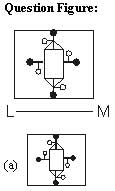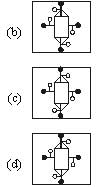49. How many triangles are there it the following figure ?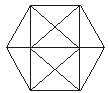(a) 20
(b) 24
(c) 28
(d) 32

50. From the given answer figures, select the one in which the question figure is hidden/embedded.